# NCERT Solutions For Class 10 Maths Chapter 6 Triangles Ex 6.4

Get Free NCERT Solutions for Class 10 Maths Chapter 6 Ex 6.4 PDF. Triangles Class 10 Maths NCERT Solutions are extremely helpful while doing your homework. Exercise 6.4 Class 10 Maths NCERT Solutions were prepared by Experienced ncert-books.inTeachers. Detailed answers of all the questions in Chapter 6 Maths Class 10 Triangles Exercise 6.4 provided in NCERT TextBook.

You can also download the free PDF of Ex 6.4 Class 10 Triangles NCERT Solutions or save the solution images and take the print out to keep it handy for your exam preparation.

## NCERT Solutions For Class 10 Maths Chapter 6 Triangles Ex 6.4

NCERT Solutions for Class 10 Maths Chapter 6 Triangles Ex Ex 6.4 are part of NCERT Solutions for Class 10 Maths. Here we have given NCERT Solutions for Class 10 Maths Chapter 6 Triangles Exercise 6.4

Question 1.
Let ∆ABC ~ ∆DEF and their areas be, respectively, 64 cm2 and 121 cm2. If EF = 15.4 cm, find BC.
Solution:Question 2.
Diagonals of a trapezium ABCD with AB || DC intersect each other at the point O. If AB = 2 CD, find the ratio of the areas of triangles AOB and COD.
Solution:Question 3.
In the given figure, ABC and DBC are two triangles on the same base BC. If AD intersects BC at O, show that:$\frac { ar\left( ABC \right) }{ ar\left( DBC \right) } =\frac { AO }{ DO }$Solution: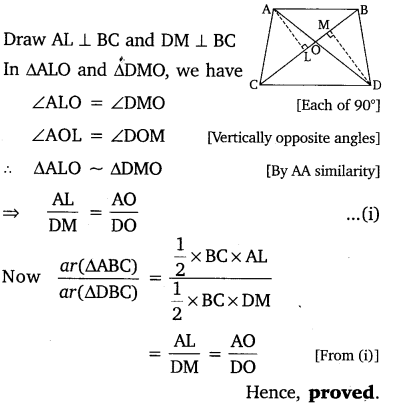Question 4.
If the areas of two similar triangles are equal, prove that they are congruent.
Solution: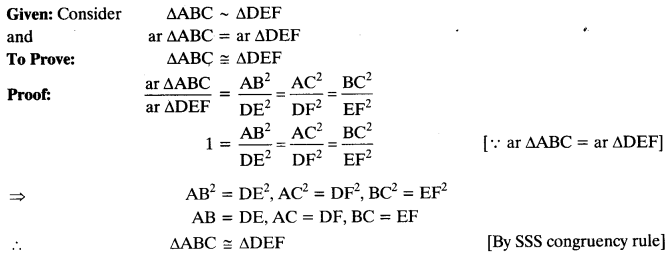Question 5.
D, E and F are respectively the mid-points of sides AB, BC and CA of ∆ABC. Find the ratio of the areas of ∆DEF and ∆ABC.
Solution: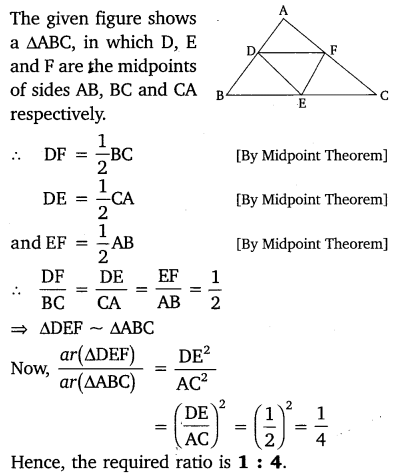Question 6.
Prove that the ratio of the areas of two similar triangles is equal to the square of the ratio of their corresponding medians.
Solution: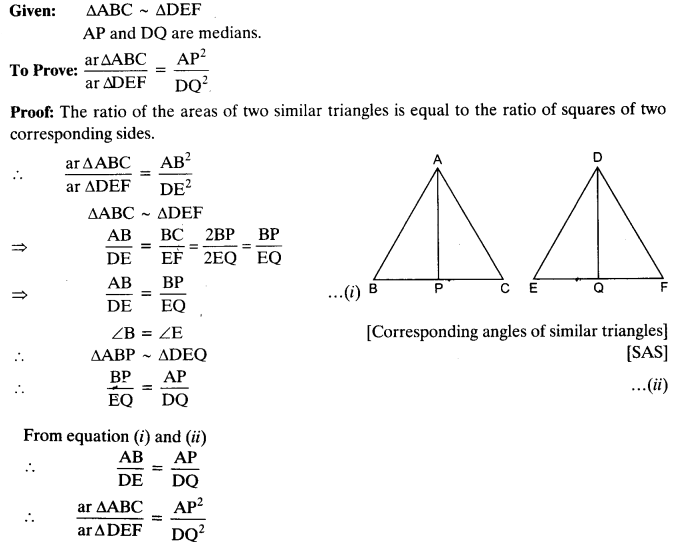Question 7.
Prove that the area of an equilateral triangle described on one side of a square is equal to half the area of the equilateral triangle described on one of its diagonals.
Solution: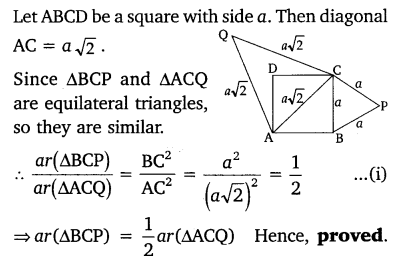Question 8.
Tick the correct answer and justify
(i) ABC and BDE are two equilateral triangles such that D is the mid-point of BC. Ratio of the areas of triangles ABC and BDE is
(a) 2 :1
(b) 1:2
(c) 4 :1
(d) 1:4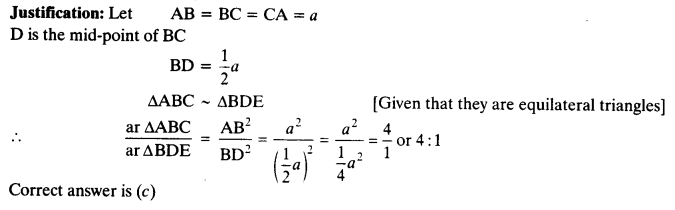(ii) Sides of two similar triangles are in the ratio 4 : 9. Areas of these triangles are in the ratio
(a) 2 : 3
(b) 4 : 9
(c) 81 : 16
(d) 16 : 81error: Content is protected !!
+